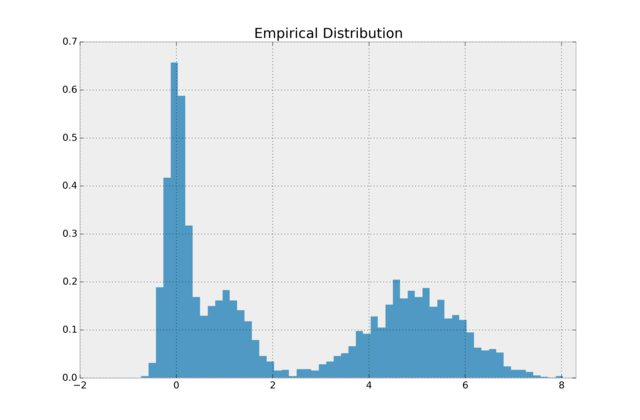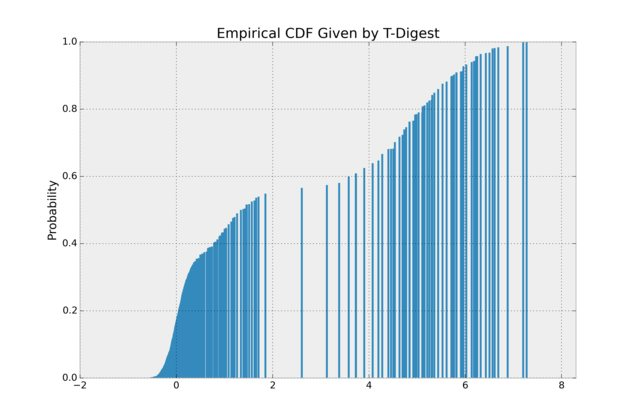# Percentile and Quantile Estimation of Big Data: The t-Digest

Posted by Cameron Davidson-Pilon on

Suppose you are interested in the sample average of an array. No problem you think, as you create a small function to sum the elements and divide by the total count.

Next, suppose you are interested in the sample average of a dataset that exists on many computers. No problem you think, as you create a function that returns the sum of the elements and the count of the elements, and send this function to each computer, and divide the sum of all the sums by the sum of all the counts.

Next, suppose you are interested in the sample median of that same distributed dataset. No problem you think, as you create a function that sorts the array and takes the middle element, and send this function to each computer, and - wait. That won't work.

The problem is is that the median of median is not equal to the median. This is why many SQL engines do not support a MEDIAN function (which left many analysts scratching their heads). What's needed is an algorithm that can approximate the median, while still being space efficient.

## Introducing the t-Digest

First published in 2013 by the uber-practical and uber-intelligent Ted Dunning, the t-Digest is a probabilistic data structure for estimating the median (and more generally any percentile) from either distributed data or streaming data. Internally, the data structure is a sparse representation of the cumulative distribution function. After ingesting data, the data structure has learned the "interesting" points of the CDF, called centroids. What do I mean by that? Consider the following very lopsided distribution:(It's really a mixture of Normals). The data has empirical CDF:The t-Digest will literally eat this dataset, and try to represent the "interesting" points of its cumulative distribution. We can plot the internal sparse representation of the CDF:The vertical lines denote where the digest thinks "interesting and relevant" centroids are. This is really neat. We can see the digest has only kept the parts of the CDF where the CDF is changing fastest. This makes sense: these are where quantities like the percentile are changing quickest. Flat portions of the CDF, like near x=3 and x=8, only need to be summarized by a few points.

## Size Considerations

Running a small test locally, I streamed 8mb of pareto-distributed data into a t-Digest. The resulting size was 5kb, and I could estimate any percentile or quantile desired. Accuracy was on the order of 0.002%.

## Associative and Commutative Properties of the t-Digest

So we can stream in data to the t-Digest, but how can we use this data structure for map-reduce? In the map stage, we can process data into the t-Digest sequentially, so we have N t-Digests in N computers. At the reduce stage, we can define an addition operation on two t-Digests as follows. Create a new t-Digest and treat the internal centroids of the two left-hand side digests as incoming data. The resulting t-Digest is a only slightly larger, but more accurate, t-Digest. Amazing: the t-Digest literally eats itself.

## t-Digest in Python

Interested in the algorithm, but without any code to read (I can't yet read Ted's implementation in Java), I wrote a semi-efficient t-Digest in Python (with helpers from Cython). You can find it in the CamDavidsonPilon/tdigest repo. There are some further optimizations to do, but it's probably efficient enough for most offline work!

## Conclusion

I highly recommend Dunning's original paper on the t-Digest below - it's an easy read. Him and some other authors are constantly improving the data structure as well, see issues here

## References

1. Dunning, T. "COMPUTING EXTREMELY ACCURATE QUANTILES USING t-DIGESTS". https://github.com/tdunning/t-digest/blob/master/docs/t-digest-paper/histo.pdf
2. Original t-Digest implementation
3. Python t-Digest implementation

## Latest Data Science screencasts available

• Your article on t-digest is so clearly and well written, that I thought you might like to know there is an update to t-digest, published today: https://arxiv.org/abs/1902.04023
- Ellen

Ellen Friedman on
• Reading this later than the others but found it very informative, not to mention logical. Great service posting this and the contribution by welch. Thanks!

marty rose on
• Cameron, your explanation of t-digest is beautifully clear – very well written and illustrated. Thank you for making this available. EF

Ellen Friedman on
• Excellent problem statement & explanation.

Rajasekar on
• A fast javascript implementation: https://www.npmjs.com/package/tdigest

Like you, I based this on a reading of the paper, finding the reference Java implementation to be a challenging read. A great speedup can be had by caching the cumulative distribution weights and not updating them with every insertion, at the cost of some harmless imprecision in the occupancy/splitting calculation.

welch on